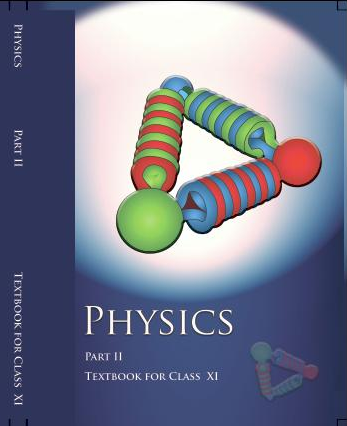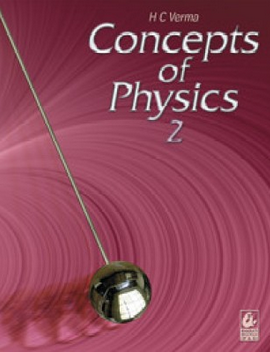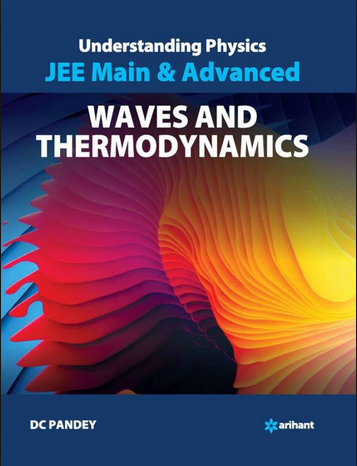# Kinetic theory of Gases (Weightage 3%)   Share

### Topics from Kinetic theory of Gases

• Kinetic theory of gases- assumptions, concept of pressure (13 concepts)
• Equation of state of a perfect gas, work done on compressing a gas (2 concepts)
• Kinetic energy and temperature:rms speed of gas molecules: Degreesof freedom (9 concepts)
• Applications to specific heat capacities of gases: Mean free path, Avogadro'snumber (8 concepts)
• States of matter (1 concepts)
• Ideal gas, its equation and laws of ideal gas (5 concepts)
• Real gas (1 concepts)
• Pressure of an ideal gas (1 concepts)
• Maxwell's law (3 concepts)
• Law of equipartition of energy (4 concepts)

### Important Books for Kinetic theory of Gases

•••Exams
Articles
Questions